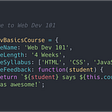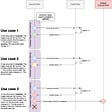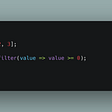# Introduction to memoization in JavaScript

## How to optimize a function by avoiding computing the same things several times

Memoization is a technique for storing values returned by a function to avoid having to redo computations that have already been performed previously.

This technique is especially very useful when we have a function which is called regularly and when its computation is expensive.

We assume here that we are working with pure functions. In particular, they must always return the same value for the same list of arguments: we can therefore store computed values for later use, thus allowing us to bypass a redundant computation.

# A simple example

A naive implementation of a function that returns the nth number in the Fibonacci sequence would be written as follows:

`const fib = n => n < 2n ? n : fib(n - 1n) + fib(n - 2n);`

Note: in this example, we are working with objects of type `bigint` . So do not be surprised by the presence of literal values suffixed by the character `n` which indicates that we are working with`bigint` and which has nothing to do with the parameter `n`! In what follows, we will sometimes omit this character for brevity.

This implementation is problematic. Let’s take a look at the computations performed when calling `fib(5)`:

`fib(5): fib(4) + fib(3)fib(4): fib(3) + fib(2)fib(3): fib(2) + fib(1)fib(2): fib(1) + fib(0)`

We observe that some values are computed several times and that we could have avoided 5 of the 9 significant recursive calls (ignoring the `fib(0)` and `fib(1)` calls which are anyway less expensive than cache-related computations).

We can avoid this problem by setting up a cache that would store the computed values for later use:

`const memoFib = n => {  const cache = [];  const fib = n => {    if (n in cache) {      return cache[n];    }    if (n < 2n) {      return n;    }    return cache[n] = fib(n - 1n) + fib(n - 2n);  };  return fib(n);};`

This solution consists in creating a closure on a cache array in which we store the returned values corresponding to function calls on arguments that have not already been encountered in our recursive calls.

In this optimized version, the `memoFib(5)` call performs the following computations:

`fib(5): fib(4) + fib(3), cache: [,, fib(2), fib(3), fib(4), fib(5)]fib(4): fib(3) + fib(2), cache: [,, fib(2), fib(3), fib(4)]fib(3): fib(2) + fib(1), cache: [,, fib(2), fib(3)]fib(2): fib(1) + fib(0), cache: [,, fib(2)]`

The values displayed in bold correspond to the values retrieved from the cache and therefore there are no subsequent recursive calls from there. Thus, our optimized version only makes 4 recursive calls instead of the previous 9, and the gap widens as the value of `n` grows.

# A generic solution

## Introduction

In this section, we will write a `memoize` function so that we can write the optimized `memoFib` function in the following concise way:

`const memoFib = memoize(fib);`

Since we want to write a generic solution, and therefore a solution accepting a function that can take any number of arguments, we must keep in mind that we add an additional cost to our function corresponding to cache-related computations. Thus, memoization generates an additional cost even if we can hope that the gain obtained from it will be greater: everything is a question of compromise. After all, if memoization was free, it would be implemented by default and you would not have to worry about computing the same values several times.

## Solution

We first need to find a mechanism that allows us to check whether the arguments encountered when calling a function have already been encountered during a previous call. If this is done easily using the `===` operator when it comes to primitive values, what do we do when it comes to references? The `===` operator in this case allows us to check if two objects have the same reference but not if two objects with different references are indeed similar. So we need a function that allows us to compare two objects differently.

We can do this by creating primitive values from our objects. For example, a hash function would allow us to do this easily and concisely:

`const memoize = fn => {  const cache = {};  return (...args) => {    const key = JSON.stringify(args);    if (key in cache) {      return cache[key];    }    return cache[key] = fn(...args);  };};`

The hash function used depends on your needs but in this example code, we suggest simply using `JSON.stringify` to create a hash of the arguments passed to our function.

Note that it is a good practice to add a `hash` parameter to our`memoize` function: this way, we could create our own memoization function by choosing a hash function `hashFn` of our choice, optionally with a default value:

`const memoizeFactory = (hashFn = JSON.stringify) =>  fn => {    const cache = {};    return (...args) => {      const key = hashFn(args);      if (key in cache) {        return cache[key];      }      return cache[key] = fn(...args);    };  };`

Note: `JSON.stringify` would not work with our `fib` function because it does not know how to serialize objects of type `bigint` (type introduced with the ES2020 specification). Since we have only one parameter that consists of a primitive value in our `fib` function, our hash function will simply return the first argument from the argument list.

# Using our memoization function

We can now easily create and then use our `memoize` function to try to optimize our initial`fib` function:

`import memoizeFactory from './memoizeFactory';const memoize = memoizeFactory(args => args);const fib = n => n < 2n ? n : fib(n - 1n) + fib(n - 2n);const memoFib = memoize(fib);`

If you have tested this new memoized function, you may have noticed that it gives unfortunately no significant performance gain compared to our previous function. Indeed, if some of the following calls of the `memoFib` function will be faster, the internal recursive calls always refer to the unmemoized function`fib` rather than `memoFib`!

In the next section, we will explore two other ways that allow us to obtain a memoized function almost equivalent to the function that we have memoized manually.

# Memoization of recursive calls within a function

The problem being that recursive calls make use of the unmemoized function, a natural solution would be to memoize the function when defining it:

`const fib = memoize(n => n < 2n ? n : fib(n - 1n) + fib(n - 2n));`

We thus obtain the correctly memoized version since `fib` now directly refers to the memoized version.

But what if we import an unmemoized recursive function from another package? This should not happen to you, but for fun, let’s try writing a memoization function that takes an existing recursive function and takes into account its recursive calls.

It looks like the only way to solve this problem would be to rewrite the recursive function as we have done above. Fortunately, it is possible to do this automatically using some JavaScript metaprogramming features.

Here is what a solution to our problem might look like:

We will not go into a lengthy explanation of how this function works here. Just remember that the idea behind it is to dynamically generate a function on the same model as the one we have immediately memoized when defining it.

# Performance analysis

Now that we have a first acceptable solution, we need to check that memoization actually brings a performance gain. If this seems obvious in this case, it is not always the case and we must therefore measure the gain associated with memoization.

We can write the following performance test showing the execution time of the call to each of our functions for `n = 42`:

`const testFib = logger => n => ([label, fib]) => {  const startFib = performance.now();  const val = fib(n);  const endFib = performance.now();  const duration = (endFib - startFib).toFixed(4);  logger(`[ \${ duration }ms ] -> \${ val } : \${label}`);};const myTest = testFib(console.log)(42n);`

Next, let’s assume that we want to test three functions `fib`, `manualMemoFib` and `memoFib` defined in the three ways seen previously (without cache, with manual cache and finally by using the `memoizeFactory` function generalized to recursive functions). We can then run the `myTest` function on each of these three functions:

`[  ['default implementation', fib],  ['manually memoized', manualMemoFib],  ['automatically memoized', memoFib]].forEach(myTest);`

We obtain the following results:

`[ 38967.3392ms ] -> 267914296 : default implementation[ 0.0744ms ] -> 267914296 : manually memoized[ 0.1563ms ] -> 267914296 : automatically memoized`

That’s exactly what we wanted: we wrote the `fib` function in an expressive and familiar way without losing too much performance thanks to our memoization function!

--

--

--

## More from DailyJS

JavaScript news and opinion.

## GSoC 2022 | Score Lab | Week 1 | React Email project updates## NextJS + Rust Twitter Streaming With Websocket## Web Dev 101: Course Kickoff## Build your own Function Retry Mechanism with Realm## Releasing Skclusive-UI 2.0 for Blazor## How to implement a custom column search in React material-table with Django REST Framework## DevOps JavaScript — Intro to Writing Scripts With zx## What is Abstraction in Object Oriented Programming?## Advanced Functions: Function Objects, NFE## JavaScript/TypeScript Array Filter## Introduction to RxJS